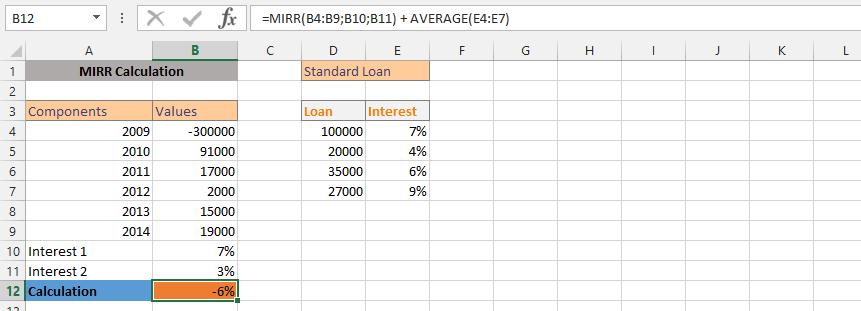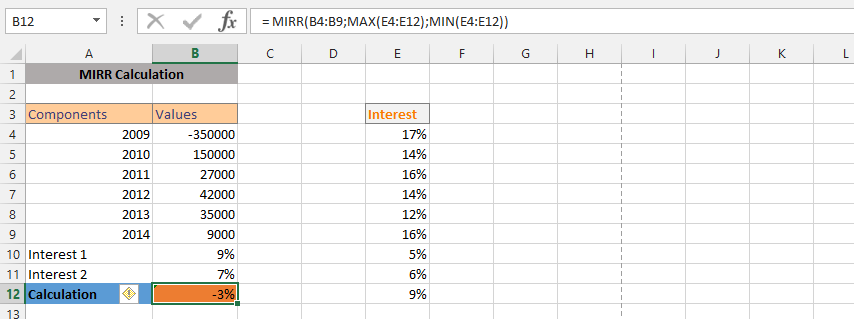### MIRR Function In Excel

MIRR (Modified Internal Rate of Return) term is basically used in financial management or corporate finance and it indicates the profitability of an investment and therefore is commonly used in when making business decision, when choosing between investments. Calculation of MIRR uses a series/schedule of payments (which includes an initial outflow, along with the net income/inflows), in calculation of MIRR compounding of interest is done with the assumption of Net Present Value of the investment is zero.

The major difference between the MIRR and IRR (Internal Rate of Return) is that, in MIRR calculation, it considers the initial cost of the investment and also the interest received on the reinvestment of cash (compounding), whereas the IRR calculation does not consider the compounding effect.

MIRR is an improved version of their IRR approach to capital budgeting decisions. It does not require the assumption that the project cash flows are reinvested at the IRR; rather, it factors in a discrete reinvestment rate into the model.

Decision rule: MIRR of projects greater the hurdle rate (of project) should be accepted; in case of mutually exclusive projects, project with higher MIRR should be selected.

Note: in calculation of MIRR cash flows should be occur at regular intervals, but do not have to be the same amounts for each interval.

### Syntax of MIRR function in Excel:

=MIRR( range, finance_rate, reinvestment_rate )

Parameters:

• range – is a range of cells that represent the series of cash flows, (investment and net income values) that occur at regular periods. These must contain at least one negative value (representing payment) and at least one positive value (representing income)
• finance_rate – is the interest rate that you pay on the cash flow amounts.
• reinvestment_rate – is the interest rate that you receive on the cash flow amounts as they are reinvested.

### Example – choosing between two projects

Suppose you are a financial manager at some investment company and working for an engineering firm. Two of the company’s recent bids are accepted. First is related to construction of a new railroad and the second one is related to construction of a motorway. Both the projects are expected to take 3 years. The applicable financing rate is 10% and the project’s cash flows (in dollars) are given below:Both projects had positive net present values.

You are asked to recommend which project the company should accept. You believe that the economy might slow down a little in next few years and a lower reinvestment rate should be factored in. Your boss asked you to calculate MIRR for both the projects.

MIRR function is used to calculate MIRR for both projects as shown in the spreadsheet below. It uses finance rate of 10% and reinvestment rate of 8%.The motorway project should be preferred based on MIRR approach.

### A simple MIRR Formula

A client is considering an investment, and came to the broker to find out the interest it would worth after five years. Traditionally, we’d just inform the client. But, the client is persistent, and is determined to acknowledge the information by himself. For that reason, we’d setup the MIRR for him to see the details.### A More Detailed Formula

The client wants to experiment a little about the calculation, and for that reason, it became necessary to try and use the detailed information for acknowledgement of the potential interest for the details.### Flexibility in MIRR

With the information, the bank is determine the interest value, if the interest rate was specific. MIRR was the perfect and logic option for acknowledging how the whole thing would work out, and with the knowledge that the client already know how much she has invested, and how it was affecting their current financial situation. They are considering how they would easily make more money, if the interest rate was specific.### MIRR and Average

The company wants to evaluate a policy, which also includes looking at the average performance of the whole investment. This is why we are going to use both average and MIRR functions for the acknowledgement.### Average and MIRR

The situation is quite different in this case, which is under the circumstances that the client has been layout, and wants to know what works best for the client that has established different ways, but this time we would like to know how the rate has been layout for the past year, which has led to a productive data, but we do not know. This is why we will use the AVERAGE and MIRR formulas in one formula.### MIN and MIRR

The business has a long history of different rates, and this has been for quite a while. For years, we have different rates, and now we are choosing the minimum rate for the circumstances, which is why we are now using both Min and MIRR formulas.### What is MIRR of 350,000?

We have a client who has 350000, and she is trying to find out how much that money would worth, when the interest rate has already been acknowledged. This is what makes it possible to acknowledge that we need to format the cell to currency after the formula has been inputted.### IF and MIRR

The client is asking a question, which includes us acknowledging that we need to find a way to see how the performance would work. The client asks for the interest rate on investment, and we’d know that the client must have a specific amount in the investment account for that MIRR to be possible, which is why we are using both IF and MIRR formula to find answers.### MAX and MIRR

The company is evaluating how to make use of the information that is currently in the business, and we want to rather use the max and MIRR formulas to find answers. All our interest rates have been layout, but we rather use the max formula.### MIRR, MAX and MIN

In this example, the situation is very different, and we do not make an investment and interest rate that would work effectively to find the balance between the client and the business. This is where MIRR, MAX and MIN would work together to provide the perfect result.## Template

```Further reading: